# C++ Program to Calculate Student Grade

In this tutorial you will learn about the C++ Program to Calculate Student Grade and its application with practical example.

In this tutorial, we will learn to create a c++ program that will Calculate Student Grade using c++ programming

## Prerequisites

Before starting with this tutorial we assume that you are best aware of the following C++ programming topics:

• Basic C++ programming.
• Operators.
• Basic input/output.
• Basic mathematics calculation.
• Precedence.

## C++ Program to Calculate Student Grade .

In this program we will Calculate Student Grade. We would first declared and initialized the required variables. Next, we would prompt user to input Subject  numbers. Later we will find the grade of that student.

## Output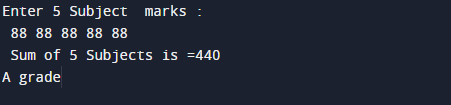In the above program, we have first declared and initialized a set variables required in the program.

• m = holds value of math.
• p = holds value of physics.
• c = holds value of chemistry
• h = holds value of Hindi.
• e= holds value of English.
• total = calculate sum of value.
• per= it will hold percentage.

In the next statement user will be prompted to enter the five subject marks  which will be assigned to variable ‘m’,’p’,’c’,’h’,’e’.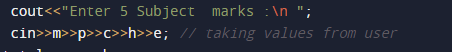after that we add all  given values  in variable total ( total =m+p+c+h+e ).Now, we calculated the percentage (per=total/5).Grades must be calculated based on following conditions.
If percentage > 85 then A grade.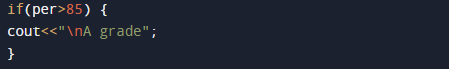elseIf (percentage < 85 && percentage >= 75) print B grade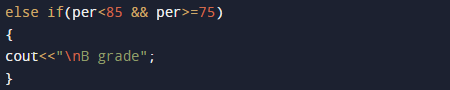else If percentage < 75 && percentage >= 50 print C grade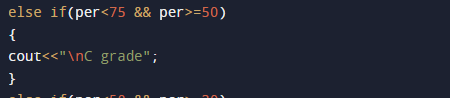else If percentage > 30 && percentage <= 50 print D grade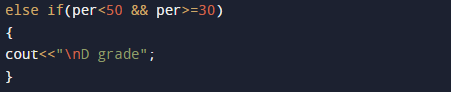else percentage <30 print fail.
First we take subject marks of  student as inputted we find the total and percentage.and after that for grade compare the percentage with different criteria as mentioned in the program.

In this tutorial we have learn about the C++ Program to Calculate Student Grade and its application with practical example. I hope you will like this tutorial.